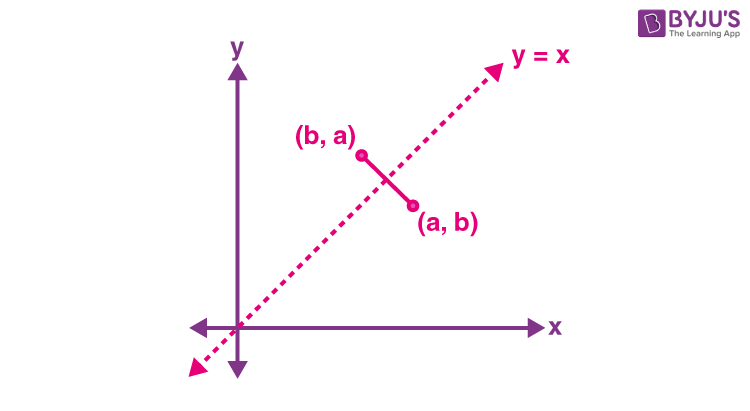Defend yourself better by mastering the science of immunity and vaccines. Secure your free spot, now! Defend yourself better by mastering the science of immunity and vaccines. Secure your free spot, now!

# Inverse Relation

In Set theory, a relation R from A to B, where A and B are non-empty sets, is a subset of the Cartesian product of A and B, i.e. A × B. Here, the set of all first elements of the ordered pairs of R is called the domain of the relation, whereas the second element set is called the range of the relation R. Also, the entire set B is referred to as the codomain of R, and we know that range is always a subset of the codomain. In maths, we can come across different types of relations. In this article, you will learn about inverse relations using graphical representation and examples.

## Inverse Relation Definition

The inverse of a relation is a relation obtained by interchanging or swapping the elements or coordinates of each ordered pair in the relation. Inverse relation in sets can be defined using the ordered pairs. The domain and range of an inverse relation can be written by swapping the domain and range of that relation. That means the domain of relation will be the range of its inverse, and the range of relation will be the domain of its inverse.

Mathematical Definition of Inverse Relation:

Suppose R is a relation of the form {(x, y): x ∈ A and y ∈ B} such that the inverse relation of R is denoted by R-1 and R-1 = {(y, x): y ∈ B and x ∈ A}. If R is from A to B, then R-1 is from B to A. In other words, if (x, y) ∈ R, then (y, x) ∈ R-1 and vice versa. Also, we know that relation in sets is a subset of the Cartesian product of sets, i.e. R is a subset of A x B, and R-1 is a subset of B x A.

## Inverse Relation Graph

If an equation describes the relation in the variables x and y, the equation of the inverse relation is obtained by replacing every x in the equation with y and every y in the equation with x. Their graphs are mirror images over the line of reflection y = x. Consider an ordered pair (a, b) of a relation expressed in terms of an equation in x and y, such that the inverse relation of this equation contains an ordered pair as (b, a) about the reflection on y = x as shown in the below figure.Let’s understand more about inverse relation with an example below.

### Inverse Relation with Example

Question:

Find the inverse of a relation R represented by {(-15, -4), (-18, -8), (-6, 2), (-12.55, 3)} and write the domain and range of the inverse relation.

Solution:

Given,

R = {(-15, -4), (-18, -8), (-6, 2), (-12.55, 3)}

Domain = {-15, -18, -6, -12.55}

Range = {-4, -8, 2, 3}

Inverse of R = R-1 = {(-4, -15), (-8, -18), (2, -6), (3, -12.55)}

Domain of R-1 = {-4, -8, 2, 3}

Range of R-1 = {-15, -18, -6, -12.55}